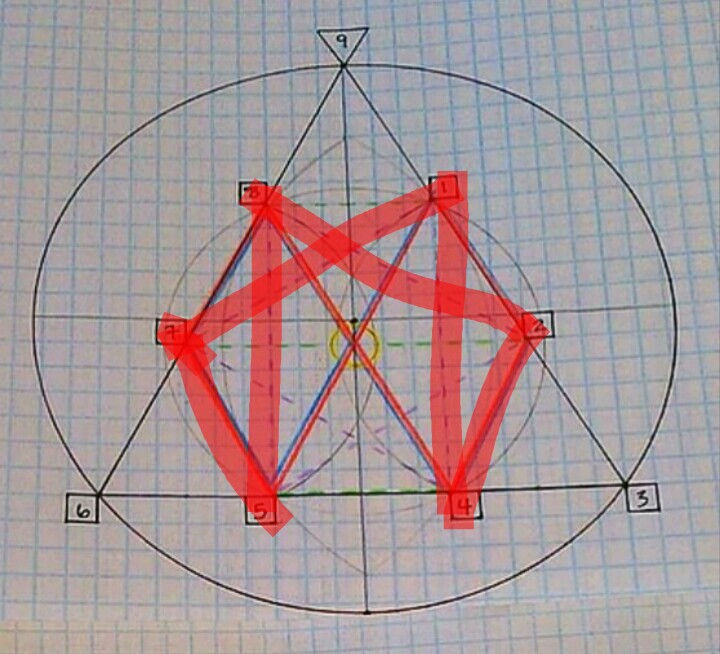top of page
Search

# Tetragrammaton tetractys enneagram tree of life 142857 superstring theory part 133/part 4

Updated: Nov 29, 2020

Tetragrammaton+tetractys+124875+enneagram+(tree of life):Tetractys=enneagram=142857=7

The numbers 1,2,4,8,7,5 and 1,4,2,8,5,7 are encoded in the tetractys tetragrammaton enneagram tree of life which encodes harmonics

The cosmic tetractys tetragrammaton enneagram tree of life which is the fruit of life encodes all superstrings

Enneagram=(star tetrahedron)=(tree of life)=tetragrammaton=tetractys=(120-cell)=(infinite dodecahedron star fractal)=(infinite tetrahedron grid)=(infinite dimensional E8 lie group)

The tetractys is energy and waves